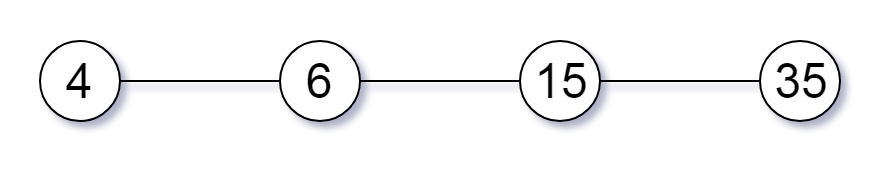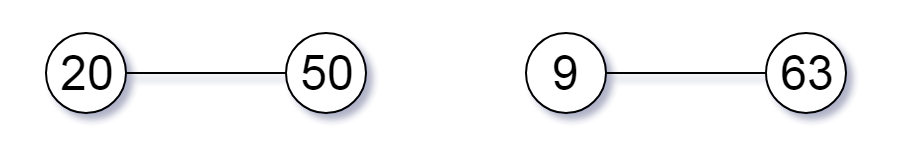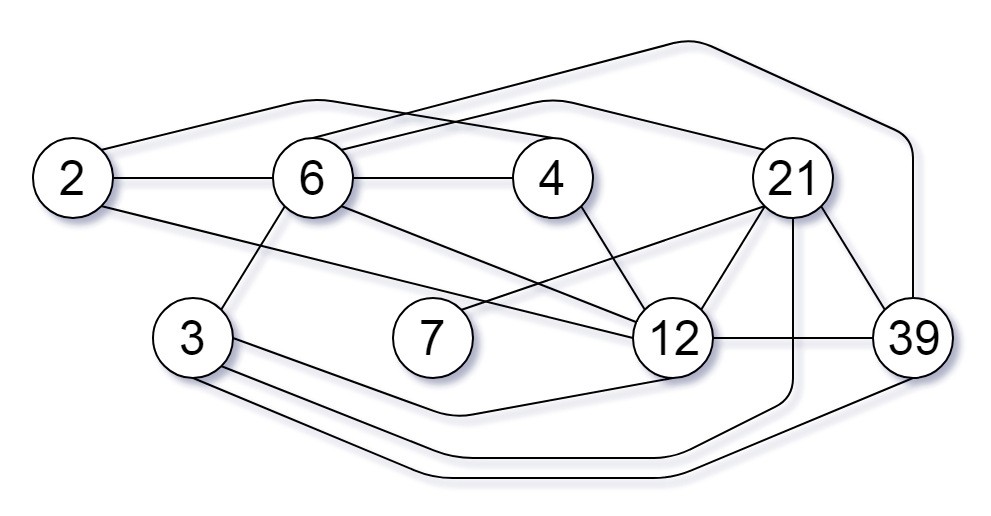All contents and pictures on this website come from the Internet and are updated regularly every week. They are for personal study and research only, and should not be used for commercial purposes. Thank you for your cooperation.

# 952. Largest Component Size by Common Factor

Given a non-empty array of unique positive integers `A`, consider the following graph:

• There are `A.length` nodes, labelled `A` to ```A[A.length - 1];```
• There is an edge between `A[i]` and `A[j]` if and only if `A[i]` and `A[j]` share a common factor greater than 1.

Return the size of the largest connected component in the graph.

Example 1:

```Input: [4,6,15,35]
Output: 4```

Example 2:

```Input: [20,50,9,63]
Output: 2```

Example 3:

```Input: [2,3,6,7,4,12,21,39]
Output: 8```

Note:

1. `1 <= A.length <= 20000`
2. `1 <= A[i] <= 100000`

Hard

Normal